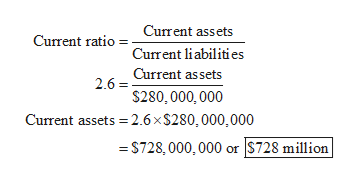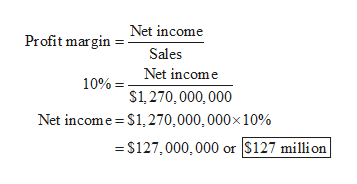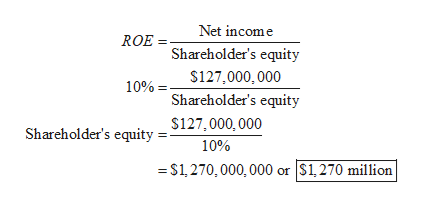Current ratio = 2.6 timesProfit margin = 10%Sales = \$1,270mROE = 10%Long-term debt to Long-term debt and equity = 60%Use the above information to complete the balance sheet below. (Enter your answers in millions.)   Current assets?millionCurrent liabilities\$280millionFixed assets?millionLong-term debt?million   Stockholders’ equity?millionTotal assets?millionTotal liabilities and equity?million

Question

Current ratio = 2.6 times
Profit margin = 10%
Sales = \$1,270m
ROE = 10%
Long-term debt to Long-term debt and equity = 60%

Use the above information to complete the balance sheet below. (Enter your answers in millions.)

 Current assets ? million Current liabilities \$280 million Fixed assets ? million Long-term debt ? million Stockholders’ equity ? million Total assets ? million Total liabilities and equity ? million

Step 1

Calculate the current assets as follows:help_outlineImage TranscriptioncloseCurrent assets Current ratioCurrent liabilities 2.6 Current as sets \$280, 000, 000 Current assets 2.6x\$280,000,000 =S728, 000,000 or \$728 million fullscreen
Step 2

Calculate the net income as follows:help_outlineImage TranscriptioncloseProfit marginNet income Sales 10% Net income S1,270,000,000 Net income \$1,270,000, 000x 10% =S127,000,000 or \$127 million fullscreen
Step 3

Calculate the shareholder’s eq...help_outlineImage TranscriptioncloseNet income ROE Shareholder's equity S127,000,000 10%= Shareholder's equity Shareholder's equity - S127,000, 000 10% S1,270,000,000 or S1,270 million fullscreen

Want to see the full answer?

See Solution

Want to see this answer and more?

Our solutions are written by experts, many with advanced degrees, and available 24/7

See Solution
Tagged in